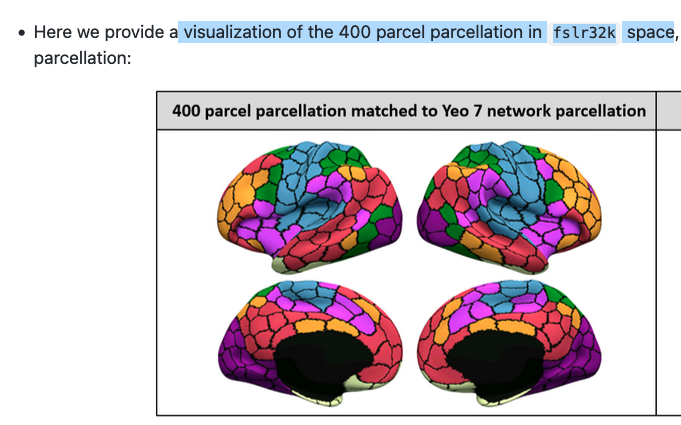# How to plot the AAL atlas to the fsl 32k surface?

I have some results obtained using as an atlas the AAL 90 region atlas.

I want to plot my results or let’s say the AAL atlas on the FSL 32k surface. Here is an example of the desired plot I am trying to make.In theory, if I have a vector with size 32k that encodes values for the 32k vertices in the FSL surface that would be enough.

I have found this 32k vector with values for the Schaefer atlas but I have no clue how to modify it to make it match my AAL. In theory, I need to build a 32k vector with values in the range 1 to 90 for my AAL atlas.

Is there a way to do that? Is there a 32k vector for the AAL atlas? Is there a correspondence between the AAL atlas and the Schaefer atlas?

Hi @makaros622,

Have you considered just projecting your image to a surface, as described in this nilearn tutorial or this one?

Best,
Steven

Unfortunately, both fail.

Here is the atlas I am trying to plot: infant-neo-aal.nii - Google Drive

`vol_to_surf` seems to be the needed function but when used on a label image the results are not good.

``````from nilearn import datasets
from nilearn import plotting

fsaverage = datasets.fetch_surf_fsaverage()

stat_img = '/Users/makaros/Desktop/infant-neo-aal.nii'
texture = surface.vol_to_surf(stat_img, fsaverage.pial_left)

fig = plotting.plot_surf_stat_map(
fsaverage.infl_right, texture, hemi='right', cmap='jet',
title='Surface right hemisphere', colorbar=True,
threshold=-1, bg_map=fsaverage.sulc_right
)
fig.show()``````

Hi @makaros622 I think nilearn.plotting.plot_surf_roi might be more appropriate here. See these examples: Loading and plotting of a cortical surface atlas - Nilearn

Also I think `vol_to_surf` will project the image onto the surface but will not put it in the same space so first you also need to make sure that your atlas is in the correct space. Nilearn does not provide registration tooling.

I found this repository DCBC/parcellations at main · DiedrichsenLab/DCBC · GitHub with the 32k vector AAL atlas and the FSL 32k surface and used this snippet to produce the following plot:

``````from nilearn import plotting
from nilearn import surface
import matplotlib.pyplot as plt

atlas = './AAL.32k.L.label.gii'

plotting.plot_surf_roi(
'./fs_LR.32k.L.inflated.surf.gii',
roi_map=parcellation,
hemi='left',
view='lateral',
darkness=.5
)
plt.show()
``````

Hope this helps!

1 Like

Thanks a lot this is indeed very useful.

Do you happen to know how I can register a nifti file to the desired 32k fls surface? So that I bring my atlas to the surface space.